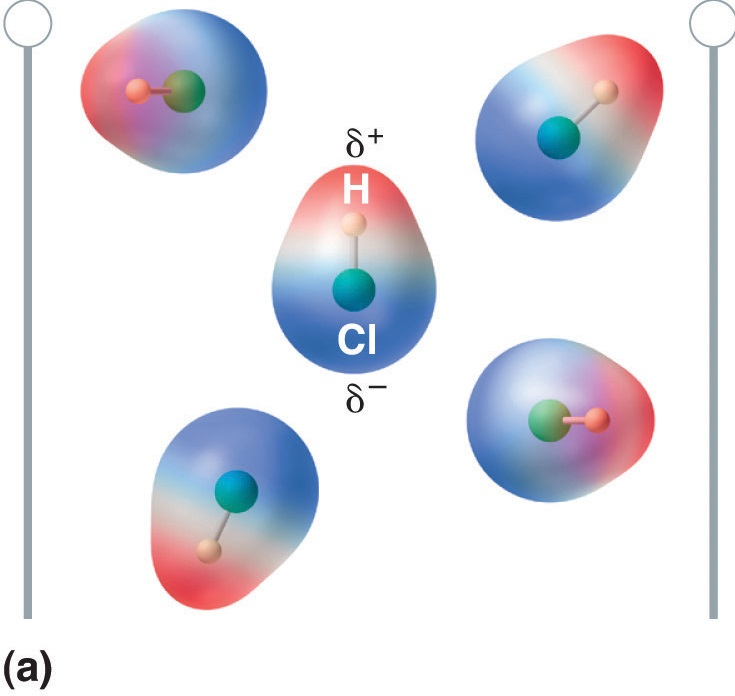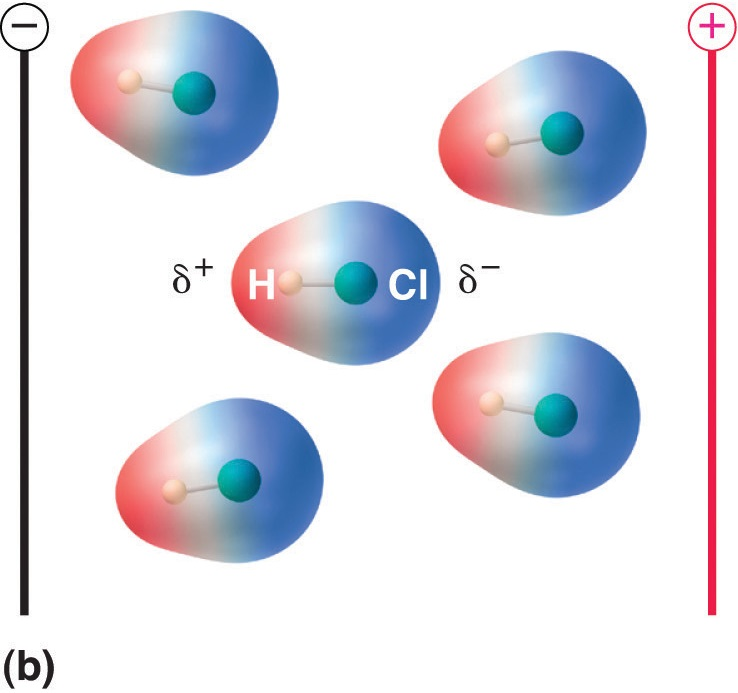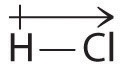# 4.5 Electronegativity, Bond Polarity, and Molecular Polarity

$$\newcommand{\vecs}{\overset { \rightharpoonup} {\mathbf{#1}} }$$ $$\newcommand{\vecd}{\overset{-\!-\!\rightharpoonup}{\vphantom{a}\smash {#1}}}$$$$\newcommand{\id}{\mathrm{id}}$$ $$\newcommand{\Span}{\mathrm{span}}$$ $$\newcommand{\kernel}{\mathrm{null}\,}$$ $$\newcommand{\range}{\mathrm{range}\,}$$ $$\newcommand{\RealPart}{\mathrm{Re}}$$ $$\newcommand{\ImaginaryPart}{\mathrm{Im}}$$ $$\newcommand{\Argument}{\mathrm{Arg}}$$ $$\newcommand{\norm}{\| #1 \|}$$ $$\newcommand{\inner}{\langle #1, #2 \rangle}$$ $$\newcommand{\Span}{\mathrm{span}}$$ $$\newcommand{\id}{\mathrm{id}}$$ $$\newcommand{\Span}{\mathrm{span}}$$ $$\newcommand{\kernel}{\mathrm{null}\,}$$ $$\newcommand{\range}{\mathrm{range}\,}$$ $$\newcommand{\RealPart}{\mathrm{Re}}$$ $$\newcommand{\ImaginaryPart}{\mathrm{Im}}$$ $$\newcommand{\Argument}{\mathrm{Arg}}$$ $$\newcommand{\norm}{\| #1 \|}$$ $$\newcommand{\inner}{\langle #1, #2 \rangle}$$ $$\newcommand{\Span}{\mathrm{span}}$$$$\newcommand{\AA}{\unicode[.8,0]{x212B}}$$

Skills to Develop

• To define electronegativity and bond polarity
• To calculate the percent ionic character of a covalent polar bond

The electron pairs shared between two atoms are not necessarily shared equally. For example, whereas the bonding electron pair is shared equally in the covalent bond in $$Cl_2$$, in $$NaCl$$ the one valence electron is stripped from the Na atom and is incorporated into the electronic structure of the Cl atom - and the compound is most accurately described as consisting of individual $$Na^+$$ and $$Cl^-$$ ions (ionic bonding). For most covalent substances, their bond character falls between these two extremes. As demonstrated below, the bond polarity is a useful concept for describing the sharing of electrons between atoms within a covalent bond:

• A nonpolar covalent bond is one in which the electrons are shared equally or nearly equally between two atoms.
• A polar covalent bond is one in which one atom has a greater attraction for the electrons than the other atom. If this relative attraction is great enough, then the bond is an ionic bond.

## Percent Ionic Character of a Covalent polar Bond

The two idealized extremes of chemical bonding: (1) ionic bonding—in which one or more electrons are transferred completely from one atom to another, and the resulting ions are held together by purely electrostatic forces—and (2) covalent bonding, in which electrons are shared equally between two atoms. Most compounds, however, have polar covalent bonds, which means that electrons are shared unequally between the bonded atoms. Figure $$\PageIndex{4}$$ compares the electron distribution in a polar covalent bond with those in an ideally covalent and an ideally ionic bond. Recall that a lowercase Greek delta ($$\delta$$) is used to indicate that a bonded atom possesses a partial positive charge, indicated by $$\delta^+$$, or a partial negative charge, indicated by $$\delta^-$$, and a bond between two atoms that possess partial charges is a polar bond.Figure $$\PageIndex{4}$$: The Electron Distribution in a Nonpolar Covalent Bond, a Polar Covalent Bond, and an Ionic Bond Using Lewis Electron Structures. In a purely covalent bond (a), the bonding electrons are shared equally between the atoms. In a purely ionic bond (c), an electron has been transferred completely from one atom to the other. A polar covalent bond (b) is intermediate between the two extremes: the bonding electrons are shared unequally between the two atoms, and the electron distribution is asymmetrical with the electron density being greater around the more electronegative atom. Electron-rich (negatively charged) regions are shown in blue; electron-poor (positively charged) regions are shown in red.

One method for calculating the percent ionic character of a bond is to use the following equation:

% ionic character = $$\dfrac{EN_{higher} - EN_{lower}}{EN_{higher}} \times 100$$

≥ 50% means the bond is ionic

> 5% but < 50% means the bond is polar covalent

≤ 5% means the bond is pure (non-polar) covalent

Table of Pauling ElectronegativitiesExample $$\PageIndex{1}$$

What is the % ionic character of a P-S bond?

Solution

Exercise $$\PageIndex{1}$$

Add texts here. Do not delete this text first.

## Bond Polarity

The polarity of a bond—the extent to which it is polar—is determined largely by the relative electronegativities of the bonded atoms. Electronegativity (χ) was defined as the ability of an atom in a molecule or an ion to attract electrons to itself. Thus there is a direct correlation between electronegativity and bond polarity. A bond is nonpolar if the bonded atoms have equal electronegativities. If the electronegativities of the bonded atoms are not equal, however, the bond is polarized toward the more electronegative atom. A bond in which the electronegativity of B (χB) is greater than the electronegativity of A (χA), for example, is indicated with the partial negative charge on the more electronegative atom:

$\begin{matrix} _{less\; electronegative}& & _{more\; electronegative}\\ A\; \; &-& B\; \; \; \; \\ ^{\delta ^{+}} & & ^{\delta ^{-}} \end{matrix} \label{8.4.1}$

One way of estimating the ionic character of a bond—that is, the magnitude of the charge separation in a polar covalent bond—is to calculate the difference in electronegativity between the two atoms: Δχ = χB − χA.

To predict the polarity of the bonds in Cl2, HCl, and NaCl, for example, we look at the electronegativities of the relevant atoms: χCl = 3.16, χH = 2.20, and χNa = 0.93. Cl2 must be nonpolar because the electronegativity difference (Δχ) is zero; hence the two chlorine atoms share the bonding electrons equally. In NaCl, Δχ is 2.23. This high value is typical of an ionic compound (Δχ ≥ ≈1.5) and means that the valence electron of sodium has been completely transferred to chlorine to form Na+ and Cl ions. In HCl, however, Δχ is only 0.96. The bonding electrons are more strongly attracted to the more electronegative chlorine atom, and so the charge distribution is

$\begin{matrix} _{\delta ^{+}}& & _{\delta ^{-}}\\ H\; \; &-& Cl \end{matrix}$

Remember that electronegativities are difficult to measure precisely and different definitions produce slightly different numbers. In practice, the polarity of a bond is usually estimated rather than calculated.

Bond polarity and ionic character increase with an increasing difference in electronegativity.

As with bond energies, the electronegativity of an atom depends to some extent on its chemical environment. It is therefore unlikely that the reported electronegativities of a chlorine atom in NaCl, Cl2, ClF5, and HClO4 would be exactly the same.

## Dipole Moments

The asymmetrical charge distribution in a polar substance such as HCl produces a dipole moment where $$Qr$$​ in meters (m). is abbreviated by the Greek letter mu (µ). The dipole moment is defined as the product of the partial charge Q on the bonded atoms and the distance r between the partial charges.

When a molecule with a dipole moment is placed in an electric field, it tends to orient itself with the electric field because of its asymmetrical charge distribution (Figure $$\PageIndex{4}$$).Figure $$\PageIndex{5}$$: Molecules That Possess a Dipole Moment Partially Align Themselves with an Applied Electric Field. In the absence of a field (a), the HCl molecules are randomly oriented. When an electric field is applied (b), the molecules tend to align themselves with the field, such that the positive end of the molecular dipole points toward the negative terminal and vice versa.

The measured dipole moment of HCl indicates that the H–Cl bond has approximately 18% ionic character (0.1811 × 100), or 82% covalent character. Instead of writing HCl as

$\begin{matrix} _{\delta ^{+}}& & _{\delta ^{-}}\\ H\; \; &-& Cl \end{matrix}$

we can therefore indicate the charge separation quantitatively as

$\begin{matrix} _{0.18\delta ^{+}}& & _{0.18\delta ^{-}}\\ H\; \; &-& Cl \end{matrix}$

Our calculated results are in agreement with the electronegativity difference between hydrogen and chlorine χH = 2.20; χCl = 3.16, χCl − χH = 0.96), a value well within the range for polar covalent bonds. We indicate the dipole moment by writing an arrow above the molecule. Mathematically, dipole moments are vectors, and they possess both a magnitude and a direction. The dipole moment of a molecule is the vector sum of the dipoles of the individual bonds. In HCl, for example, the dipole moment is indicated as follows:The arrow shows the direction of electron flow by pointing toward the more electronegative atom.

The charge on the atoms of many substances in the gas phase can be calculated using measured dipole moments and bond distances. Figure $$\PageIndex{6}$$ shows a plot of the percent ionic character versus the difference in electronegativity of the bonded atoms for several substances. According to the graph, the bonding in species such as NaCl(g) and CsF(g) is substantially less than 100% ionic in character. As the gas condenses into a solid, however, dipole–dipole interactions between polarized species increase the charge separations. In the crystal, therefore, an electron is transferred from the metal to the nonmetal, and these substances behave like classic ionic compounds. The data in Figure $$\PageIndex{6}$$ show that diatomic species with an electronegativity difference of less than 1.5 are less than 50% ionic in character, which is consistent with our earlier description of these species as containing polar covalent bonds. The use of dipole moments to determine the ionic character of a polar bond is illustrated in Example $$\PageIndex{2}$$.Figure $$\PageIndex{6}$$: A Plot of the Percent Ionic Character of a Bond as Determined from Measured Dipole Moments versus the Difference in Electronegativity of the Bonded Atoms.In the gas phase, even CsF, which has the largest possible difference in electronegativity between atoms, is not 100% ionic. Solid CsF, however, is best viewed as 100% ionic because of the additional electrostatic interactions in the lattice.

## Summary

Bond polarity and ionic character increase with an increasing difference in electronegativity. The electronegativity (χ) of an element is the relative ability of an atom to attract electrons to itself in a chemical compound and increases diagonally from the lower left of the periodic table to the upper right. The Pauling electronegativity scale is based on measurements of the strengths of covalent bonds between different atoms, whereas the Mulliken electronegativity of an element is the average of its first ionization energy and the absolute value of its electron affinity. Elements with a high electronegativity are generally nonmetals and electrical insulators and tend to behave as oxidants in chemical reactions. Conversely, elements with a low electronegativity are generally metals and good electrical conductors and tend to behave as reductants in chemical reactions.

Compounds with polar covalent bonds have electrons that are shared unequally between the bonded atoms. The polarity of such a bond is determined largely by the relative electronegativites of the bonded atoms. The asymmetrical charge distribution in a polar substance produces a dipole moment, which is the product of the partial charges on the bonded atoms and the distance between them.

Modified by Tom Neils (Grand Rapids Community College)

4.5 Electronegativity, Bond Polarity, and Molecular Polarity is shared under a not declared license and was authored, remixed, and/or curated by LibreTexts.